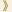HomeShaw Laureates2018Mathematical SciencesEssay
Differential equations are fundamental to large parts of mathematics, physics, and indeed all the sciences. For example, if a physical system is in a certain state, and obeys certain laws, then a differential equation will tell you the state of the system infinitesimally later, and by putting together all these infinitesimal changes one can follow the evolution of the system in time. Or if a static system is held together by certain forces, a differential equation can often say how one part of the system depends on its immediate neighbours, and putting together this local information can give a global description of the system.

The simplest differential equations, known as ordinary differential equations, concern functions that depend on just one variable. For example, if a stone is thrown vertically in the air, then there is an ordinary differential equation that describes how its height varies as a function of time, given its initial height and velocity. However, the most useful equations, known as partial differential equations, concern functions of several variables (such as, for example, three spatial coordinates and one temporal coordinate) and are significantly more complicated as a result. Partial differential equations can be used to model heat flow, fluid motion, electromagnetic waves, quantum mechanics, the shape of soap bubbles, and innumerable other physical phenomena.

A few very simple equations can be solved explicitly — that is, one can find an exact formula for their solutions — but this is very much the exception rather than the rule. Instead, one has to be content with being able to show that solutions exist, and with being able to say something about how they behave.

A very important example of this is the Navier-Stokes equation, which describes the motion of a viscous fluid. It is not known whether, given appropriate initial conditions, there must be a solution to the Navier-Stokes equation that remains well-behaved forever, or whether singularities will necessarily develop. To put it more graphically, if you stir a bucket of water, is there a danger that a week later it will blow up? Probably not, but nobody knows how to prove this, and it is one of the major unsolved problems of mathematics. Indeed, it is considered so important that it was chosen as one of the seven problems for the solution of any of which the Clay Mathematics Institute offers a reward of one million US dollars. (One of the problems has been solved, so the total number is now down to six.)

Although it is not known how to solve the Navier-Stokes equations, one can find so-called “weak solutions”, which are abstract objects that solve the equations, but not in quite the sense one wants. This very important insight goes back to Leray in 1934, who looked at a sequence of approximations to the Navier-Stokes equations, finding for each one a solution, and then constructing a limiting object that can be interpreted as solving the actual Navier-Stokes equation. However, these limiting objects are not genuine functions: if one could show that they were “regular”, then they would be, and the Navier-Stokes problem would be solved. A famous result of Caffarelli, Kohn and Nirenberg is the closest anybody has come to that: it shows that weak solutions exist that are regular except on a set of singularities that has to be very small in a precise mathematical sense.

Another area in which Caffarelli has created a new and highly influential theory is obstacle problems. Here one would like to know the shape that will be taken by an elastic membrane with a given boundary if it has to lie above a certain obstacle. The shape taken will be the one that minimizes its energy, but the important questions concern how well-behaved, or “regular”, a solution of this kind will be. As with all important problems in partial differential equations, this one arises in many contexts that at first glance look extremely different, including fluid filtration in porous media, and financial mathematics.

In general, because one does not usually have explicit formulae for solutions to partial differential equations, the analysis of their properties is very hard, and depends on extremely delicate estimates. Caffarelli is a master at this, frequently coming up with arguments that have left other researchers wondering how he could possibly have thought of them. He continues to work at the forefront of the field and has had a huge influence, both through his own work and that of his doctoral students, many of whom have themselves become extremely distinguished mathematicians. In a way that few mathematicians achieve even once, he has repeatedly created important areas almost from scratch that are extremely active to this day.

26 September 2018   Hong Kong